# Examples

Akantu’s example are separated in 2 types, the C++ and the python ones.

## C++ Examples

### Solid Mechanics Model

#### Boundary conditions usage

In predifined_bc it is shown how to impose Dirichlet boundary condition using the predefined BC::Dirichlet::FixedValue (Fig. 29). Three built-in Dirichlet functors exist: FixedValue, FlagOnly and IncrementValue.

To define another functor, a class inherited from BC::Dirichlet::DirichletFunctor can be created as illustrated in the example user_defined_bc where a sinusoidal BC is imposed. The corresponding sinusoidal displacement is depicted in Fig. 30. Note that a Neumann BC is also imposed.

#### Static test cases

In static, an example of how to solve a static problem is presented. The problem geometry is shown in Fig. 31. The left and bottom side of a 2D plate are blocked with rollers and nodes from the left side are displaced upward by $$0.01\%$$ of the length of the plate.

The solution for the static analysis is shown in Fig. 32.

#### explicit

In explicit, an example of a dynamic solution with an explicit time integration is shown. The explicit scheme is selected using the _explicit_lumped_mass constant:

model.initFull(_analysis_method = _explicit_lumped_mass);


Note that it is also the default value, hence using model.initFull(); is equivalent.

This example models the propagation of a wave in a steel beam. The beam and the applied displacement in the $$x$$ direction are shown in Fig. 33.

The length and height of the beam are $$L={10}\textrm{m}$$ and $$h = {1}\textrm{m}$$, respectively. The material is linear elastic, homogeneous and isotropic (density: $$7800\mathrm{kg/m}^3$$, Young’s modulus: $$210\mathrm{GPa}$$ and Poisson’s ratio: $$0.3$$). The imposed displacement follow a Gaussian function with a maximum amplitude of $$A = {0.01}\textrm{m}$$. The potential, kinetic and total energies are computed. The safety factor is equal to $$0.8$$.

The dynamic solution is depicted in Fig. 34.

#### implicit

In implicit, an example of a dynamic solution with an implicit time integration is shown. The implicit scheme is selected using the _implicit_dynamic constant:

model.initFull(_analysis_method = _implicit_dynamic);


This example consists of a 3D beam of $$10\mathrm{m}\times1\mathrm{m}\times1\mathrm{m}$$ blocked on one side and is on a roller on the other side. A constant force of $$5\mathrm{kN}$$ is applied in its middle. Fig. 35 presents the geometry of this case. The material used is a fictitious linear elastic material with a density of $$1000 \mathrm{kg/m}^3$$, a Young’s Modulus of $$120 \mathrm{MPa}$$ and Poisson’s ratio of $$0.3$$. These values were chosen to simplify the analytical solution.

An approximation of the dynamic response of the middle point of the beam is given by:

$u\left(\frac{L}{2}, t\right) = \frac{1}{\pi^4} \left(1 - cos\left(\pi^2 t\right) + \frac{1}{81}\left(1 - cos\left(3^2 \pi^2 t\right)\right) + \frac{1}{625}\left(1 - cos\left(5^2 \pi^2 t\right)\right)\right)$

Fig. 36 presents the deformed beam at 3 different times during the simulation: time steps 0, 1000 and 2000.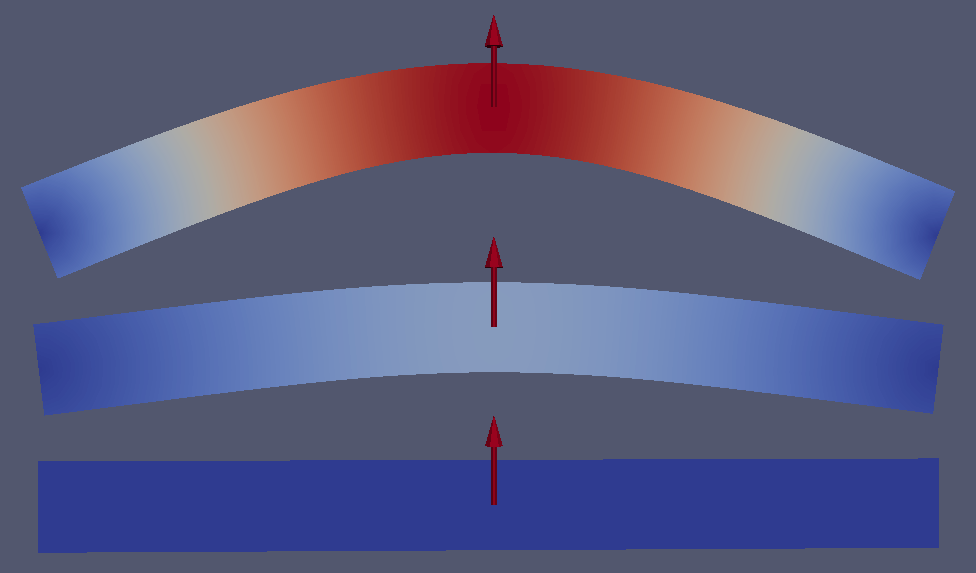Fig. 36 Deformed beam at three different times (displacement $$\times 10$$).

#### new_material

In new_material it is shown how to use a user-defined material for the simulation. All the details are described in Adding a New Constitutive Law. The geometry solved is shown in Fig. 37.

#### parser

In io/parser, an example illustrating how to parse an input file with user-defined parameters is shown. As before, the text input file of the simulation is precised using the method initialize. In the input file, additionally to the usual material elastic section, there is a section user parameters with extra user-definied parameters. Within the main function, those parameters are retrived with:

const ParserSection & usersect = getUserParser();
Real parameter_name = usersect.getParameter("parameter_name");


#### dumper

In io/dumper, examples of advanced dumping are shown.

dumpable_low_level aims at illustrating how to manipulate low-level methods of DumperIOHelper. The goal is to visualize a colorized moving train with Paraview. It is shown how to dump only a part of the mesh (here the wheels).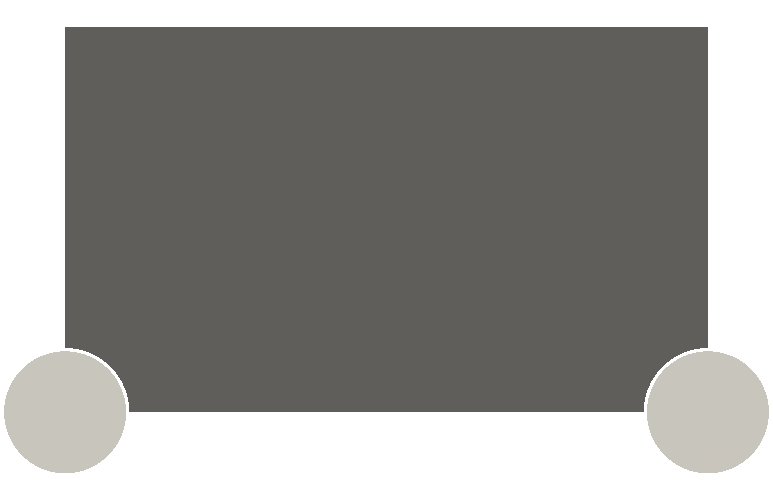Fig. 38 The wheels and the full train are dumped separately.

dumpable_interface does the same as dumpable_low_level but using dumpers::Dumpable which is an interface for other classes (Model, Mesh, …) to dump themselves.

#### parallel

In parallel, an example of how to run a parallel simulation is presented.

### Solid Mechanics Cohesive Model

Solid mechanics cohesive model examples are shown in solid_mechanics_cohesive_model. This new model is called in a very similar way as the solid mechanics model:

SolidMechanicsModelCohesive model(mesh);


Cohesive elements can be inserted intrinsically (when the mesh is generated) or extrinsically (during the simulation).

#### cohesive_intrinsic

In cohesive_intrinsic, an example of intrinsic cohesive elements is shown. The cohesive elements are inserted between $$x = -0.26$$ and $$x = -0.24$$ before the start of the simulation with model.getElementInserter().setLimit(_x, -0.26, -0.24);. Elements to the right of this limit are moved to the right. The resulting displacement is shown in Fig. 40.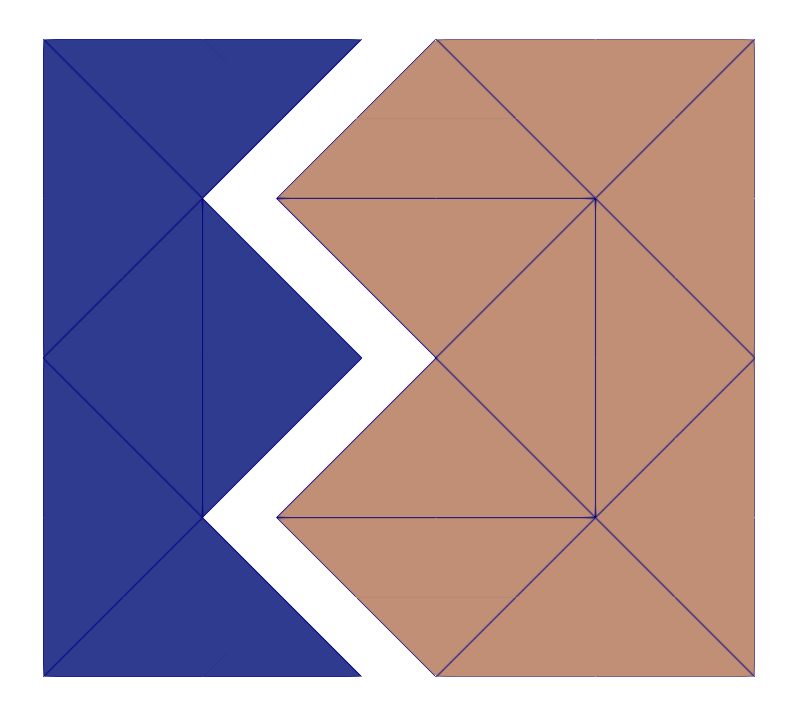Fig. 40 Displacement in the x direction for the cohesive_intrinsic example.

#### cohesive_extrinsic

In cohesive_extrinsic, cohesive elements are inserted during the simulation but at a location restricted with::

CohesiveElementInserter & inserter = model.getElementInserter(); inserter.setLimit(_y, 0.30, 0.20); model.updateAutomaticInsertion();

A displacement is then imposed based on the elements location. The corresponding displacements is shown in Fig. 41.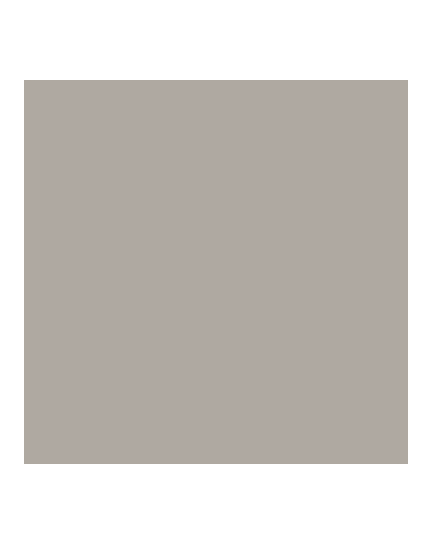Fig. 41 Displacement in the y direction for the cohesive_extrinsic example.

#### cohesive_extrinsic_ig_tg

In cohesive_extrinsic_ig_tg, the insertion of cohesive element is not limited to a given location. Rather, elements at the boundaries of the block and those on the inside have a different critical stress defined in the material.dat file. The four block sides are then moved outwards. The resulting displacement is shown in Fig. 42.

### Contact mechanics model

contact_explicit_static and contact_explicit_dynamic are solving a 2D Hertz contact patch test.

#### diffusion_model

In diffusion_model, examples of the HeatTransferModel are presented.

An example of a static heat propagation is presented in heat_diffusion_static_2d.cc. This example consists of a square 2D plate of $$1 \text{m}^2$$ having an initial temperature of $$100 \text{K}$$ everywhere but a none centered hot point maintained at $$300 \text{K}$$. Fig. 43 presents the geometry of this case (left) and the results (right). The material used is a linear fictitious elastic material with a density of $$8940 \text{kg}/\text{m}^3$$, a conductivity of $$401 \text{W}/\text{m}/\text{K}$$ and a specific heat capacity of $$385 \text{J}/\text{K}/\text{kg}$$.

In heat_diffusion_dynamics_2d.cc, the same example is solved dynamically using an explicit time scheme. The time step used is $$0.12 \text{s}$$.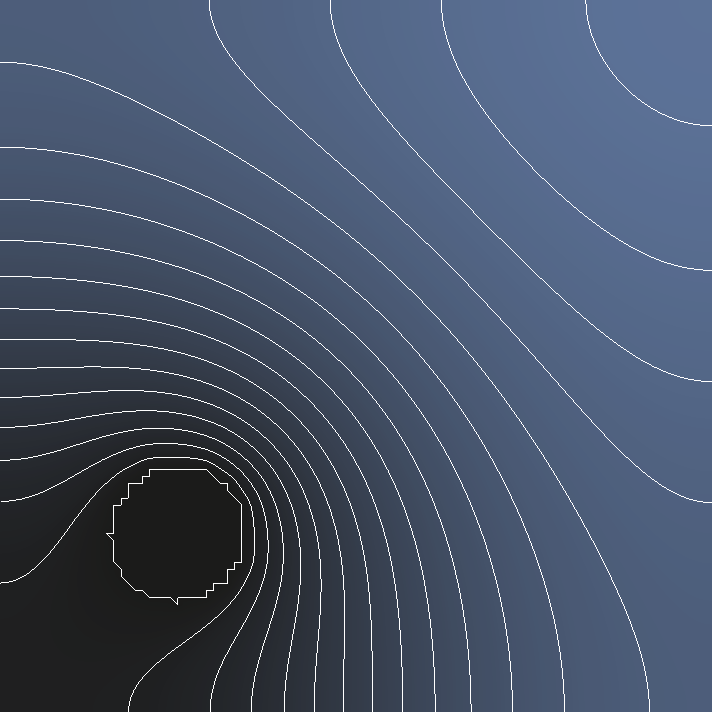Fig. 44 Temperature field after 15000 time steps = 30 minutes. The lines represent iso-surfaces.

In heat_diffusion_dynamics_3d.cc, a 3D explicit dynamic heat propagation problem is solved. It consists of a cube having an initial temperature of $$100 \text{K}$$ everywhere but a centered sphere maintained at $$300 \text{K}$$. Fig. 45 presents the resulting temperature field evolution.

### Phase-field model

#### static

phase_field_notch.cc shows hot to set a quasi-static fracture simulation with phase-field. The geometry of the solid is a square plate with a notch. The loading is an imposed displacement at the top of the plate (mode I).

In addition, this example shows how to extract clusters delimited by the mesh boundaries and elements with damage values beyond a threshold. This can be useful to extract fragments after crack propagation.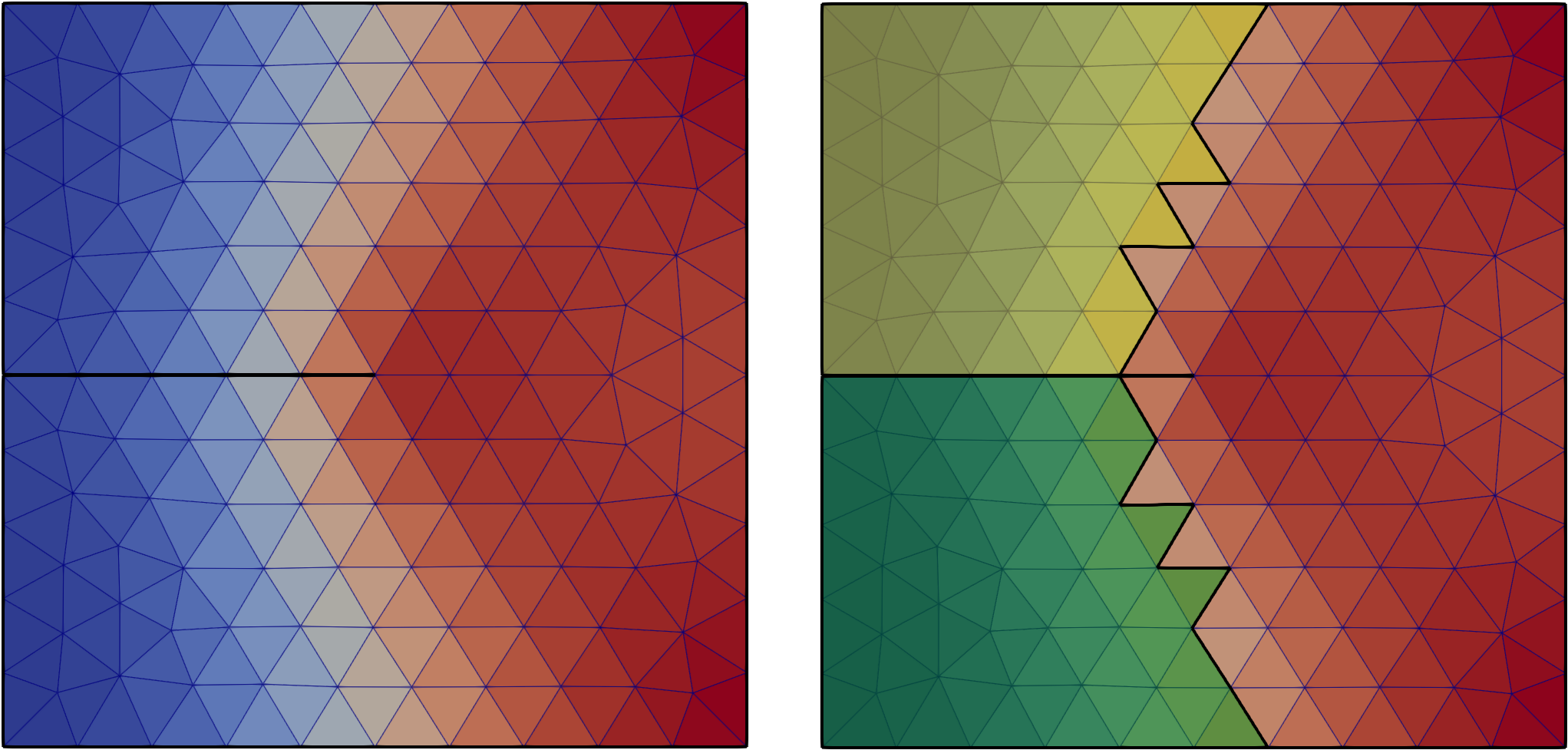Fig. 47 Damage field after a few iterations and two clusters (fragments) extracted.

## Python examples

### Solid Mechanics Model

#### dynamics

In dynamics, an example of a dynamic simulation solved with an explicit time intergration is shown. This examples model the propagation of a wave in a bar. The geometry is depicted in Fig. 48. A pulse is impose as an initial displacement over the bar. Results are depicted in Fig. 49.

#### eigen_modes

In eigen_modes it is shown how to compute the eigen modes. The mode can be specify with the -m argument. Simulation is performed on a bar where a pulse is imposed. The -p will plot the following figures Fig. 50: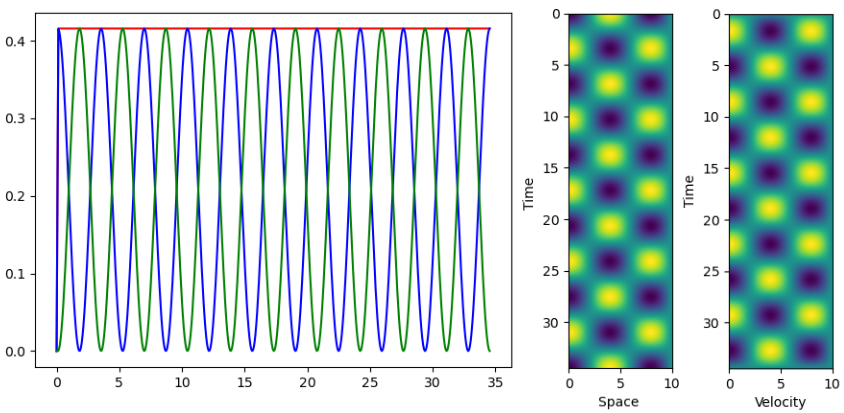Fig. 50 Energy norms as a fonction of time (left), space-time diagram for diplacements (center) and space-time diagram for velocities (right) with the default values.

#### custom-material

In custom-material.py it is shown how to create a custom material behaviour. In this example, a linear elastic material is recreated. It is done by creating a class that inherits from aka.Material and register it to MaterialFactory:

class LocalElastic(aka.Material):
[...]

def allocator(_dim, unused, model, _id):
return LocalElastic(model, _id)

mat_factory = aka.MaterialFactory.getInstance()
mat_factory.registerAllocator("local_elastic", allocator)


Wave propagation of a pulse in a bar fixed on the top, bottom and right boundaries is simulated using an explicit scheme. Results are shown in Fig. 51.

In bi-material.py, the same principle is used to create a bimaterial square. The displacement is shown in Fig. 52.

#### stiffness_matrix

stiffness_matrix shows how to get the stiffness matrix from a mesh.

#### solver_callback

In solver_callback, it is shown how to write a solver callback function. It is done by writing a class that inherit from InterceptSolverCallback:

class SolverCallback(aka.InterceptSolverCallback):
[.....]

solver_callback = SolverCallback(model)

model.solveStep(solver_callback)


In that case, the solver callback is used to modify the stiffness matrix.

### Solid Mechanics Cohesive Model

#### cohesive

In cohesive/plate.py, an example of extrinsic cohesive elements insertion is shown. This example simulates a plate with a pre-existing crack pulled. The geometry is depicted in Fig. 53.

The problem is solved statically until the onset of instability and is then solved dynamically with an explicit integration scheme. This is done by adding a new solver with model.initNewSolver():

model = aka.SolidMechanicsModelCohesive(mesh)
model.initFull(_analysis_method=aka._static, _is_extrinsic=True)
model.initNewSolver(aka._explicit_lumped_mass)
[....]
model.solveStep("static")
[....]
model.solveStep("explicit_lumped")


The crack opening is displayed in Fig. 54.

#### fragmentation

fragmentation shows an example of a 1D bar fragmentation with extrinsic cohesive elements. It uses a custom boundary condition to impose a constant velocity. This is done by creating a class that inherits from DirichletFunctor. The result is shown in Fig. 55.

### Contact mechanics model

In compression.py it is shown how to couple the Solid Mechanics Model with the Contact Mechanics Model. The example simulate the contact betweem two blocks.

### Phase-field model

#### static

phasefield-static.py shows how to setup a static phase-field fracture simulation. An imposed displacement is imposed on the top of a notched square plate.

In static simulations, we use loading steps to apply the displacement incrementally. At each time step, the solvers of the solid mechanics model and the phase-field model are called alternately until convergence is reached.